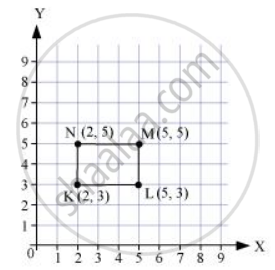Share

# Plot the Following Points on a Graph Sheet. Verify If They Lie on a Line K(2, 3), L(5, 3), M(5, 5), N(2, 5) - CBSE Class 8 - Mathematics

ConceptLinear Graphs - Location of a Point

#### Question

Plot the following points on a graph sheet. Verify if they lie on a line

K(2, 3), L(5, 3), M(5, 5), N(2, 5)

#### Solution

We can plot the given points and join the consecutive points on a graph paper as follows.Hence, points K, L, M, and N are not lying on the same line.

Is there an error in this question or solution?

#### APPEARS IN

NCERT Solution for Mathematics Textbook for Class 8 (2018 to Current)
Chapter 15: Introduction to Graphs
Ex. 15.20 | Q: 1.3 | Page no. 243
Solution Plot the Following Points on a Graph Sheet. Verify If They Lie on a Line K(2, 3), L(5, 3), M(5, 5), N(2, 5) Concept: Linear Graphs - Location of a Point.
S